# An overview of Independent Component Analysis

A

## 1. What is ICA ?

Independent Component Analysis is a technique of separating signals from their linear mixes. We could assume two signals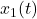andthat are a linear combination of two signals source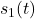and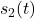, the relationships of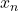and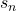are shown in the following system of linear equations where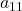,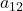,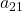and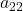are the parameters determining the mixing of the signals.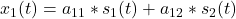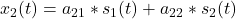These parameters(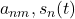) are not known, so the system of linear equations cannot be not solved by classical methods. To solve the problem, we have to use the statistical properties of the mixing signals of the independent components, which are considered as data. We assume that the source signals are statistically independent and non-Gaussian.

Q: Why statistically independent sources?
A: Ref to Central Limit Theorem.

We consider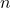observations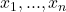fromlinear combinations ofIndependent Components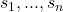. Thus, we consider the mixtures and the independent components as random variables and not as time signals.

In a compact form this could be written as: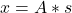where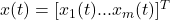,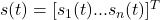, and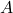is a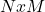matrix.

## 2. Implementation restrictions

However, the previous equation is far from reality and used only for the sake of simplification. The “real” mathematical model, which does not assume linearity and takes into account additive noise, is described by the following equation: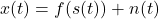Where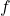: unknown transformation function and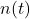: additive noise vector that “offends” the signals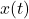Now, the purpose of ICA analysis is to reconstruct the unknown signals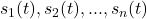, by constructing a function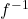, which is as close to as possible, ie the theoretically inverse function of.

## 3. Data Pre-processing

We will now explain the pre-processing performed by most ICA algorithms before actually applying ICA.

### 3.1 Centering

Subtract its mean vector to make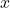a zero-mean variable.

### 3.2 Whitening

A first step in many ICA algorithms is to whiten the data. This means that we remove any correlations in the data. Why do that? A geometrical interpretation is that it restores the initial “shape” of the data. The whitening process is simply a linear change of coordinate of the mixed data. Once the ICA solution is found in this “whitened” coordinate frame, we can easily reproject the ICA solution back into the original coordinate frame. Advantage: reduce the dimensionality.

## 4 Implementation of HOS-based techniques for the Independent Component Analysis

HOS-based techniques. These, ICA algorithms are based on the construction of statistically independent components, which contain higher order statistical data.

Higher order statistics (HOS), for Gaussian distribution variables are getting zeroing. These statistical sizes are used in the ICA analysis and form the basis of a series of algorithms, the so-called HOS algorithms. It is logical, therefore, that HOS algorithms can not proceed without the non-Gaussian distribution.

One of the most known algorithm is FastICA. This algorithm is trying to maximize thefunction of kurtosis or negentropy (HOS techniques) to separate the “true” components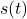from the Gaussian distribution of the mixed signals## 5. Compare ICA and PCA

PCA: Finds directions of maximal variance in gaussian data
ICA: Finds directions of maximal independence in nongaussian data

## 6. Application Examples

1. Image Separation [ LINK ]

## 7. Bibliography sources

1. Independent Component Analysis: A tutorial [ LINK ]
2. What is Independent Component Analysis: A Demo [ LINK ]
3. Independent Component Analysis for dummies [ LINK ]
4. Independent Component Analysis PPT [ LINK ]
5. Independent Component Analysis on Images [ LINK ]